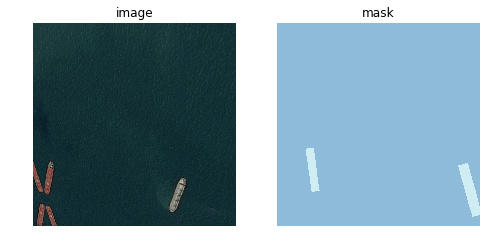# Mask isn't transformed the same way as image

Somehow the masks are transformed differently than the respective images in my implementation. Everything without transforms works fine though.

``````data = (src.transform(vision.get_transforms(), size=IMG_SHAPE, tfm_y=True)
.databunch(bs=BATCH_SIZE)
.normalize(vision.imagenet_stats))

def imshow(idx):
fig, (ax1, ax2) = plt.subplots(1, 2, figsize = (8, 4))
data.train_ds[idx].show(ax=ax1)
ax1.set_title('image')
ax1.axis('off')
data.train_ds[idx].show(ax=ax2)
ax2.axis('off')

plt.show()

imshow(6)
``````Even basic flip with `dihedral_affine` in most cases results in different images. Is there something to consider? The only difference to other examples is the way I load masks, instead of using the default `open_mask` in `SegmentationLabelList` I use a custom function to load them from run-length encodings.

``````def masks_as_image(masks, shape):
# Decode ship masks into image segments and overlay them

img_id = fn.stem + fn.suffix

classes = ['no_ship', 'ship']
src = (SegmentationItemList.from_folder(path/'train_v2')
.random_split_by_pct(0.2)
.label_from_func(lambda x: x, classes=classes))
``````

But it shouldn’t have any effect on later transformations since my mask tensors are in valid shape.

Someone else posted on this example, and it looks like the problem lies in `rle_decode`. This dataset seems to use a differnet convention than the fastai function.

That’s why I transposed the output of `rle_decode` so that mask and image perfectly overlay, so the problem may lay somewhere later in the code. I’ll try to do some more tests, such as save the mask to disk and use the default `open_mask` on it.

Hi Polakowo,

How did you transpose the output of rle_decode, external libraries kinda break the downstream processes.

Regards,
Rony